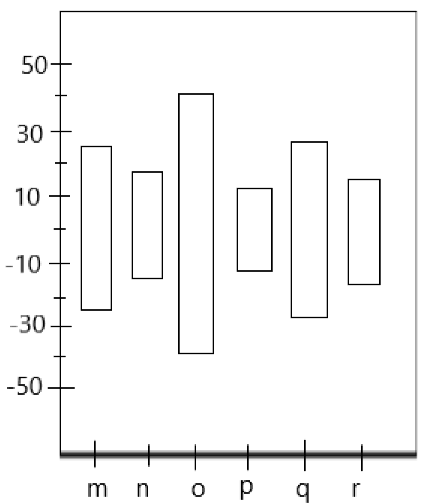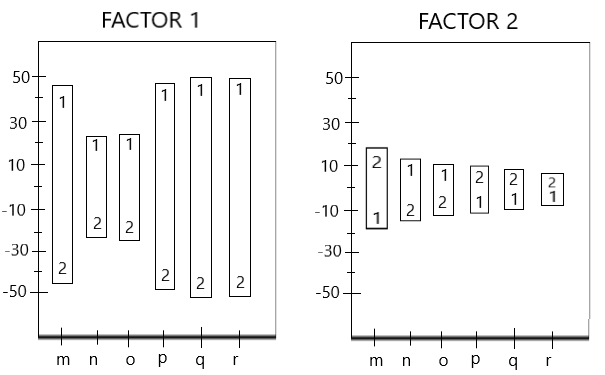Introduction to Block Plot

• Last Updated : 22 Jan, 2021

A block plot is similar to a box plot, showing the importance of a particular factor in the data. [As shown in Fig 1] Just like ANOVA, it is used to show statistical significance.FIg.1: Block Plot

Structure of Block Plot:

The structure of block plot includes:

• X-axis — All combinations of secondary variables/features from m to r.
• Y-axis — Response variable y. (significance measure)

We can use it when: data is of an extremely non-normal distribution. (Unlike ANOVA, where the data had to be of a normal distribution)

Interpreting a Block PlotFig 2: Interpreting results from a Block plot

In a block plot, we deal with levels. (the numbers 1 and 2 are levels as shown in Fig 2) We can notice the following observations:

Observation 1:

• If the levels of a block plot are uniform (i.e. all from 1->2 or 2->1), then that factor is significant to the data. [Block plot for Factor 1]
• If the levels of a block plot are non-uniform. (i.e some from 1->2 while some from 2->1), the factor under observation is not that significant. [Block plot for Factor 2]

Observation 2:

The height of the bar determines the impact of that factor on the response variable.

• The block plot for FACTOR 1 is consistently taller, hence it is of higher significance. (i.e. FACTOR 1 is important)
• The block plot for FACTOR 2 is comparatively lower, hence making it less significant. (i.e. FACTOR 2 is not that important)